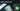# Hackerrank Solution of Simple Array Sum in Golang

August 24th, 2018
|

Solution for hackerrank warmup question simple array sum in golang. In this blog I'll discuss my solution, what mistakes I initially made and what concepts I learned.

# Problem Statement

The question can be found at this link. The problem statement basically states that we need to print the sum of an array of integers whose length is provided as input. Couple of things to note here is

• We need to take array length as input
• In the next line, we are provided with an array of numbers, whose sum we need to print
• we are solving this in golang (assuming we know how to take input in golang, refer to my last post in case you need a refresher )

# Challenges

• Choose our preferred language as golang on hackerrank. The moment we do that, we get some 50-60 lines of code which are very unfamiliar to someone who is new to language.
• To solve this problem only thing we need to know is how to take input, show output, iterate over an array(kind of), and calculate sum.

# Solution

This is the template you get on hackerrank for this problem statement.

``````package main

import (
"bufio"
"fmt"
"io"
"os"
"strconv"
"strings"
)

/*
* Complete the simpleArraySum function below.
*/
func simpleArraySum(ar []int32) int32 {
/*
*/

}

func main() {

stdout, err := os.Create(os.Getenv("OUTPUT_PATH"))
checkError(err)

defer stdout.Close()

writer := bufio.NewWriterSize(stdout, 1024 * 1024)

checkError(err)

var ar []int32

for arItr := 0; arItr < int(arCount); arItr++ {
arItemTemp, err := strconv.ParseInt(arTemp[arItr], 10, 64)
checkError(err)
arItem := int32(arItemTemp)
ar = append(ar, arItem)
}

result := simpleArraySum(ar)

fmt.Fprintf(writer, "%d\n", result)

writer.Flush()
}

if err == io.EOF {
return ""
}

return strings.TrimRight(string(str), "\r\n")
}

func checkError(err error) {
if err != nil {
panic(err)
}
}``````
• First go ahead and delete everything in the pre-existing template.
• Add a package name `package main`
• Import "fmt" library for input and output operations.
• Next add a empty main function below our import statement.
• If any of these steps seems unfamiliar to you, please refer to my last post

Up until now our code should look something like this

``````package main
import "fmt"

func main() {

}``````

Now it is time to write the program logic. First we need to declare a variable to take length of our array as input. Second, we need to take the whole array as input, or maybe not. We are only concerned with each single integer in array once, we don't need to store those numbers for any future use. We can very well take single numbers as inputs, keep them in temp variable, add that to sum and move ahead. Well that sums our logic. Next we need a sum variable to store our sum. Let's go ahead and add these variable declarations.

``var n,temp,sum int``

Next step is taking the length of the array as input. We will just use our Scan as that is easier(no formatting required).

``fmt.Scan(&n)``

Now we need to iterate n times, take inputs and calculate their sum.

# Loops in Golang

If you are coming from other programming languages, you may find it weird that golang has only for loops. It does not have while or do while loops. However there are ways you can use golang's for loop to achieve the same behaviour that while and do while provides in any other programming languages. We will use a for loop to take inputs here and find their sum.

``````for i:=0;i<n;i++{
fmt.Scan(&temp)
sum += temp
}``````

Okay, so the for loop looks little weird. So if you watched my video along with last post, you already know what `:=` means. Basically it is declaring and initializing a variable `i` which is visible only in the scope of for loop. Next we have our loop conditions and increment operator. The parenthesis `()` around the whole thing is not required, however the `{}` are must even if you only have a single statement inside for loop. Then we are taking a integer input in temp. Next We are adding that to sum.

Hold on a minute, wouldn't `sum += temp` throw a error for the first time? You never initialized the value of sum to 0. In golang it wouldn't. In golang all variables are assigned zero values when they are declared. Integers have 0 as zero values, strings have empty strings "" etc. So when we declared sum it also got initialized with 0, hence `sum += temp` won't throw an error for the first time.

Phew!!! All the heavy lifting has been done. Let's just the sum and get over with it.

``fmt.Println(sum)``

Now Your final code should look something like this.

``````package main
import "fmt"

func main(){
var n, temp,sum int
fmt.Scan(&n)
for i:=0;i<n;i++{
fmt.Scan(&temp)
sum += temp
}
fmt.Println(sum)
}``````